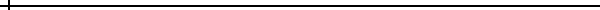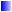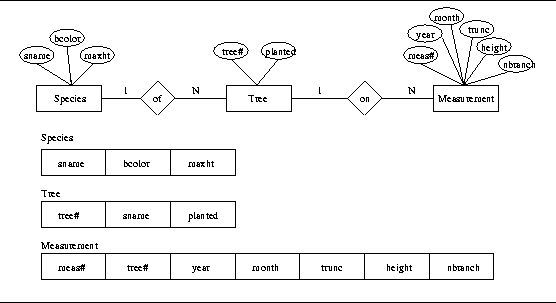# Writing Complex SQL Queries that Require Universal Quantifiers

### Jalal Kawash The University of Calgary### ABSTRACT

One major disadvantage of SQL is that the language does not provide a universal quantification construct. Queries that have universal and existential quantifiers nested and twisted are not easy to write. We illustrate, by example, a systematic way to translate tuple relational calculus queries to SQL. This is accomplished by introducing the SQL-Normal-From of tuple relational calculus from which generating SQL code is automatic. This method is very helpful for students in a course on database systems and for database practitioners.

Keywords: Database Education, SQL, Relational Calculus, Quantification, Universal, Existential Implications## 1. Introduction

SQL, the most commonly used language in commercial relational database management systems [2,5,3], has some shortcomings. One major disadvantage of SQL is its lack of a universal quantification construct. It is correct that for all and every English phrases can be satisfied in SQL through negating its existential quantifier construct EXISTS. However, when these phrases become nested and twisted with each other and with existential phrases, translating the English query to SQL may become a very subtle process.

The use of universal quantifiers to logically understand and express the for all and every phrases is more natural and more intuitive than negating existential quantifiers. The use of implications with universal quantifiers is also a natural and intuitive way to express the for all and every English phrases. Furthermore, students and programmers are accustomed to the use of if-then constructs.

In this paper, we present a systematic way to translate relational calculus expressions to SQL queries. We demonstrate the easiness of the approach by example. A sequence of well defined steps to translate relational calculus expressions to a special form, which we call the SQL-Normal-Form, make the generation of the SQL code automatic.

This method is sought to be helpful for students in a course on database systems, and for practitioners who are involved in this kind of logically twisted queries.

## 2. SQL-Normal-Form

The key idea is to rewrite tuple relational calculus expressions in a special form, which we call the SQL-Normal-Form, and abbreviate it SQLNF. Once in SQLNF, a relational calculus expression can be translated to SQL easily. The main idea in SQLNF is to eliminate universal quantifiers from the relational calculus expression. To do so, we need to recall the following equivalences where F, F1, and F2 are formulas and t is a tuple variable (The complete definitions for formulas, tuple variables, and the like are elsewhere ):Implication: (F1 => F2) <=> (!F1 | F2)Universal Quantification: (FORALL t)(F(t)) <=> (!EXISTS t)!(F(t))DeMorgan's: !(F1 & F2) <=> (!F1 | !F2) and !(F1 | F2) <=> (!F1 & !F2)Double Negation: !!F <=> F

We refer to the highlighted negation in the formula (!EXISTS t)!(F(t)) by a sandwiched negation.

Definition 2.1   A formula F is in SQLNF if it does not contain: 1. Double Negation, 2. Universal Quantification. 3. Implication, or 4. Sandwiched Negation.

Each one of these undesired forms can be eliminated by one of the equivalences stated above. In particular, the sandwiched negation can be eliminated by applying DeMorgan's (replacing (!EXISTS t)!(F(t)) by (!EXISTS t)(!F(t))).

Definition 2.2   A tuple relational calculus expression E = {t1.a1,t2.a2,..., tn.an | F(t1,t2,..., tn,tn+1,tn+2,...,tn+m)} is in SQLNF if and only if F is in SQLNF.

To put an expression E in SQLNF, we follow the following procedure.

### Normalization Procedure

1.
Eliminate all implications (by applying Implication).
2.
Eliminate all universal quantifiers (by applying Universal Quantification).
3.
Eliminate double negation (by applying Double Negations).
4.
Eliminate sandwiched negation (by applying DeMorgan's).

This process is referred to as normalization and an expression that is in SQLNF is normalized. We illustrate this normalization procedure by example.

## 3. Examples

Figure 1 depicts a simple forestry database . The database consists of three entity types (Species, Tree, and Measurement), and two relationship types (of and on).Species attributes are the species name (sname), bark color (bcolor), and maximum height (maxht). Attribute sname is Species primary attribute. Tree has the attributes tree number (tree#) and the year it was planted (planted). Its primary attributes is tree#. Measurement is described by a measurement number (meas#), year and month performed, trunk size (trunk), height, and the number of branches (nbranch). The Measurement's primary attribute is meas#. Relationship type of is 1:N relating Tree to Species. Many trees could be of a certain species. Relationship type on is 1:N relating Tree to Measurement. A tree can have many measurements performed on it.

Query 1. Get the species name of each species with red bark were one and all trees of that species were planted before 1985.

Relational Calculus Expression for Query 1
E1 = {s.sname | Species(s) & s.bcolor = RED & (FORALL t)((Tree(t) & t.sname = s.sname) => t.planted < 1985) & (EXISTS t)(Tree(t) & t.sname = s.sname & t.planted < 1985)}

Normalizing E1

1.
eliminate implications
E1 = {s.sname | Species(s) & s.bcolor = RED & (FORALL t)(!(Tree(t) & t.sname = s.sname) | t.planted < 1985) & (EXISTS t)(Tree(t) & t.sname = s.sname & t.planted < 1985)}

2.
eliminate universal quantifiers
E1 = {s.sname | Species(s) & s.bcolor = RED & (!EXISTS t)!(!(Tree(t) & t.sname = s.sname) | t.planted < 1985) & (EXISTS t)(Tree(t) & t.sname = s.sname & t.planted < 1985)}

3.
eliminate sandwiched negation
E1 = {s.sname | Species(s) & s.bcolor = RED & (!EXISTS t)(!!(Tree(t) & t.sname = s.sname) & t.planted >= 1985) & (EXISTS t)(Tree(t) & t.sname = s.sname & t.planted < 1985)}

4.
eliminate double negation
E1 = {s.sname | Species(s) & s.bcolor = RED & (!EXISTS t)((Tree(t) & t.sname = s.sname) & t.planted >= 1985) & (EXISTS t)(Tree(t) & t.sname = s.sname & t.planted < 1985)}
Query 2. Get the name and bark color for each species with a maximum height exceeding 30 meters such that every tree of that species that was planted before 1960 has had every measurement showing a height greater than 15 meters.

Relational Calculus Expression for Query2
E2 = {s.sname, s.bcolor | Species(s) & s.maxht > 30 & (FORALL t)((Tree(t) & t.sname = s.sname & t.planed < 1960) => (FORALL m)((Measurement(m) & m.tree# = t.tree#) => m.height > 15))}

Normalizing E2

1.
eliminate implications
E2 = {s.sname, s.bcolor | Species(s) & s.maxht > 30 & (FORALL t)(!(Tree(t) & t.sname = s.sname & t.planed < 1960) | (FORALL m)(!(Measurement(m) & m.tree# = t.tree#) | m.height > 15))}

2.
eliminate universal quantifiers
E2 = {s.sname, s.bcolor | Species(s) & s.maxht > 30 & (!EXISTS t)!(!(Tree(t) & t.sname = s.sname & t.planed < 1960) | (!EXISTS m)!(!(Measurement(m) & m.tree# = t.tree#) | m.height > 15))}

3.
eliminate sandwiched and double negation
E2 = {s.sname, s.bcolor | Species(s) & s.maxht > 30 & (!EXISTS t)((Tree(t) & t.sname = s.sname & t.planed < 1960) & (EXISTS m)((Measurement(m) & m.tree# = t.tree#) & m.height <= 15))}

## 4. Translation to SQL

Given an expression E is in SQLNF, the translation to SQL is straightforward. Let E = {t1.a1,t2.a2,..., tn.an | F(t1,t2,..., tn,tn+1,tn+2,...,tn+m)}. The list t1.a1,t2.a2,..., tn.an is called the left-of-| and F(t1,t2,..., tn,tn+1,tn+2,...,tn+m)} is called the right-of-|. Let Ri(t) and Rj(t) denote relations appearing in F where t is a tuple variable.

Translation Procedure

1.
The SELECT clause consists of the left-of-|.
2.
The FROM clause consists of each Ri(t) to the right-of-| such that Ri(t) is not within the scope of a quantifier.
3.
The WHERE clause consists of F translated to SQL constructs. This excludes each Ri(t) which is not within the scope of a quantifier. Since F is in SQLNF it must only consist of existential quantifiers and logical operators which in turn exist in SQL. Furthermore, for each occurrence of an Rj(t), we should expect a nested SQL statement having Rj in its FROM clause.

This procedure is best explained by example. Below are the translations of the normalized expressions.

Query 1
E1 = {s.sname | Species(s) & s.bcolor = RED & (!EXISTS t)((Tree(t) & t.sname = s.sname) & t.planted >= 1985) & (EXISTS t)(Tree(t) & t.sname = s.sname & t.planted < 1985)}

```
SELECT s.sname
FROM Species s
WHERE s.bcolor = `RED'
AND NOT EXISTS(SELECT *
FROM Tree t
WHERE t.sname = s.sname
AND t.planted >= 1985)
AND EXISTS(SELECT *
FROM Tree t
WHERE t.sname = s.sname
AND t.planted < 1985)
```

Query 2
E2 = {s.sname, s.bcolor | Species(s) & s.maxht > 30 & (!EXISTS t)((Tree(t) & t.sname = s.sname & t.planed < 1960) & (EXISTS m)((Measurement(m) & m.tree# = t.tree#) & m.height <= 15))}

```
SELECT s.sname, s.bcolor
FROM Species s
WHERE s.maxht > 30
AND NOT EXISTS(SELECT *
FROM Tree t
WHERE t.sname = s.sname
AND t.planted < 1960
AND EXISTS(SELECT *
FROM Measurement m
WHERE m.tree# = t.tree#
AND m.height <= 15))
```

### Acknowledgment

I am thankful to James Bradley for his comments on a tutorial version of this work . The example database was taken from one of his books .

## References

1
File and Database Techniques.
Holt, Rinehart, and Winston, 1982.

2
C. Date.
An Introduction to database systems.

3
R. Elmasri and S. B. Navathe.
Fundamentals of Database Systems.
Benjamin/Cummings, 1994.

4
J. Kawash.
Systematic Translation of Relational Calculus Expressions to SQL Queries: A Tutorial.
1998. Available at http://www.cpsc.ucalgary.ca/~kawash/publications.html.

5
H. F. Korth, A. Silberschatz, and S. Sudarshan.
Database System Concepts.
McGraw-Hill, 1996.Jalal Kawash
Department of Computer Science
The University of Calgary
2500 University Dr. NW